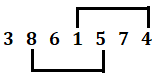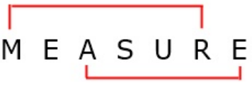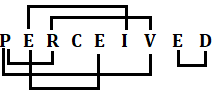Latest Banking jobs   »

# Reasoning Ability Quiz For IBPS RRB PO Prelims 2022- 8th June

Q1. How many meaningful words can be formed by using the letters ‘A, B, T and K’, once in the arrangement?
(a) 0
(b) 1
(c) 2
(d) 3
(e) None of these

Q2. If it is possible to make a meaningful word using 2nd, 4th, 6th and 8th letters of the word “MELLIFLUOUS”, then what will be the 2nd letter of that meaningful word? If no such meaningful word can be formed mark the answer as ‘X’, if two words can be formed, mark the answer as ‘Y’.
(a) L
(b) X
(c) Y
(d) U
(e) None of these

Q3. How many such pairs of digits are there in the number ‘3861574; each of which has as many digits between them as they have in mathematics in both forward and backward directions?
(a) Two
(b) One
(c) Three
(d) More than three
(e) None

Q4. How many such pair of letters are there in the word ‘MEASURE’ in which the letters are same as in the English alphabet both from backward and forward?
(a) None
(b) Two
(c) More than three
(d) Three
(e) One

Q5. In the word ‘REASONING’, if all consonants are written as their preceding letter and all vowels are written as their succeeding letters. Then how many vowels are there in the new word?
(a) None
(b) Two
(c) Three
(d) Four
(e) Five

Q6. How many such pair of letters are there in the word ‘PERCEIVED’ which have as many letters between them as they have in English alphabet series (in forward and backward both direction)?
(a) One
(b) Two
(c) Three
(d) Four
(e) More than four

Q7. If in the number ‘64793258’, 1 is subtracted from first four digits of the number and 1 is added to last four digits of the number, then which amongst the following digits is/are repetitive?
(a) Only 3
(b) Only 6
(c) 3 and 5
(d) 3 and 6
(e) None of these

Q8. How many seven-letter meaningful words (Ending with E) can be formed from the word PERFUMELESS?
(a) Five
(b) Two
(c) Four
(d) Three
(e) None of these

Q9. If in a code COLD becomes AMJB and FILE becomes DGJC then what will JUDICIARY be in that code?
(a) HSBGAGYOW
(b) HSAGAGYPW
(c) HSBGAGYPX
(d) HSBGAGYPW
(e) None of these

Q10. If the digits in the number ‘42168674’ are arranged in the descending order from left to right then what will be the product of the digits which are 3rd from left end and 4th from right end in new arrangement?
(a) 24
(b) 48
(c) 36
(d) 28
(e) 16

Q11. How many meaningful English words can be formed with all the letters of ‘ITGLYU’ using each letter only once in each word?
(a) Three
(b) None
(c) One
(d) More than three
(e) Two

Q12. How many such pairs of letters are there in the word ‘HALLOW’ each of which has as many letters between them as in the English alphabet (Both forward and backward)?
(a) None
(b) One
(c) Two
(d) Three
(e) More than three

Q13. If it is possible to make only one meaningful word with the first, second, fourth, and sixth letter of the word ‘STARVING’, which would be the second letter of that meaningful word from the right end? If more than one meaningful word can be formed, give X as the answer. If no meaningful word can be formed, give K as your answer.
(a) T
(b) S
(c) X
(d) I
(e) K

Q14. In a certain code, TROLLS is written as RULPSM. How would GROWNS be written in that code language?
(a) SORHWP
(b) RHPSOW
(c) RHWPSO
(d) PSOWHR
(e) None of these

Q15. If a meaningful word beginning with R, can be formed using all the letters of TRRPOE, then fifth letter of that word is your answer. If more than one such word can be formed then ‘M’ is your answer. If no such word is formed, then ‘N’ is your answer.
(a) O
(b) R
(c) P
(d) M
(e) N

Solutions

S1. Ans. (a)
Sol. No meaningful word is formed with the letter ‘A, B, T and K’.

S2. Ans. (c)
Sol. 2nd, 4th, 6th and 8th letters of the given word are E, L, F and U.
So, two words can be formed – FLUE, FUEL

S3. Ans. (a)
Sol. Given Number – 3861574S4. Ans. (b)
Sol.S5. Ans. (a)
Sol. As per conditions, all consonants are written as their preceding letter and all vowels are written as their succeeding letters.
R E A S O N I N G – Q F B R P M J M F; We can see in new word, there is no vowel. Hence, required answer is none.

S6. Ans. (e)
Sol. Total pairs in PERCEIVED are more than four in both directions.S7. Ans. (d)
Sol. Given Number – 64793258
If 1 is subtracted from first four digits of the number and 1 is added to last four digits of the number – 53684369
Clearly, 3 and 6 are repetitive.

S8. Ans. (a)
Sol. Meaningful words ending with E = Perfume, Perfuse, Presume, Repulse, Supreme

S9. Ans. (d)
Sol. All the letters of COLD and FILE moved two places backward as in English alphabet. So, the word JUDICIARY is written as HSBGAGYPW.

S10. Ans. (a)
Sol. Digits in descending order = 87664421
3rd from left end = 6 and 4th from right end = 4
So, product = 6×4 = 24

S11. Ans. (c)
Sol. Only one word can be made from ITGLYU = GUILTY

S12. Ans. (a)
Sol. There is no pair.

S13. Ans. (d)
Sol. Specified letters are: S, T, R and I
Meaningful word: STIR
Hence second letter from the right end is I.

S14. Ans. (c)
Sol. First and the second letters are interchanged, third and the fourth letters are interchanged and then fifth and sixth letters are interchanged in the given word. Then letters at the even places are interchanged with their immediate succeeding letter to obtain the corresponding codes.
So, GROWNS = RHWPSO

S15. Ans. (b)
Sol. Meaningful words using T, R, R, P, O and E = Porter and Report
Word beginning with R = Report
So, 5th letter of the word = R#### Congratulations!